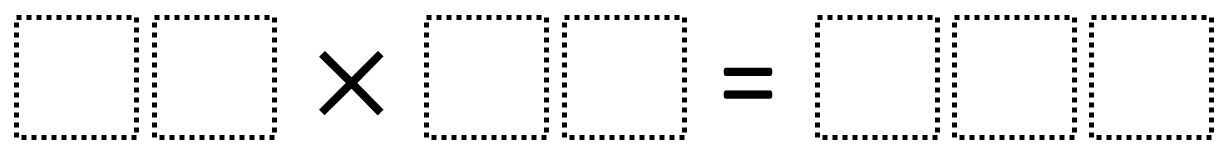# Multi-Digit Multiplication 2

Directions: Using the digits 0 to 9 at most one time each, place a digit in each box to create a true equation with the greatest possible product.### Hint

What two-digit numbers would be too low/high that when multiplied together would not make a three-digit product?

The greatest possible product found so far is 54 x 18 which equals 972 but there may be one even greater. Let us know in the comments what you find.

Source: Robert Kaplinsky

## Equivalent Expressions with Fractions

Directions: Using the digits 0 to 9 at most one time each and choosing either …

1.We found a higher product. 78 x 12 = 936

2.I found a higher product. 78 x 96 = 7488

•My student found 13 x 74 = 962

•Would this be ok because your answer has digits that were used in the problem.

3.My student and I found 18 x 54 =972 oh yea! We loved this one!

4.i fond a higher number 50 x 18=950

5.We found 74×13=962

•My 5th grade students solved this! 🙂

6.We found a solution of 83X12=996

7.Our class at FLEXSchool also got 972 = 18 x 54

•Jaydon found 54*18=972 at Underwood School in Newton.

8.Our class at FLEXSCHOOL got 972 = 18 x 54 again, the very next period.

9.my amazing other class at FLEXSCHOOL also got 972 = 18 x 54 again, the very next period. sO HAHAHA INTERNET!

10.68 x 14 = 952 —- solved by one of my 5th grade students

11.78×12=936 and I loves it very fun

12.You used 3 eights and your product was a 4 digit number. It was supposed to be a 3 digit number.

13.52 x 18 = 936

14.This is so fun! I gave this to my students for a Friday Fun Challenge and these are the answers that we got without repeating digits…
936
962
972
910

15.68 x 14 = 952

16.My fifth graders found 78 x 12 = 936

17.Ms. Smiths Kindergarten class

Nice problem but my kindergarteners found 68 x 14 = 952

18.19.20.•